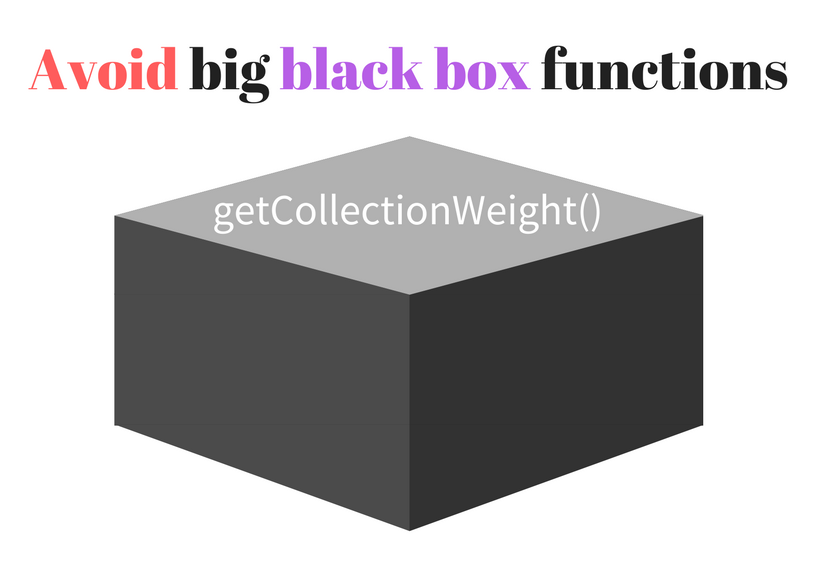# 编写小而美函数的艺术

• 函数冗长，做了太多事情
• 函数有副作用并且很难理解和调试排错
• 含糊的函数/变量命名
• 代码脆弱，一个小改动会意外地破坏应用的其它组件
• 缺乏测试的覆盖## 函数需要“小”

• `null` 或者 `undefined` 计为 `1`
• 基础类型的数据计为 `2`
• 对象或者函数类型的数据计为 `4`

### Step 0: 最初的庞大函数

``````function getCollectionWeight(collection) {
let collectionValues;
if (collection instanceof Array) {
collectionValues = collection;
} else if (collection instanceof Map) {
collectionValues = [...collection.values()];
} else {
collectionValues = Object.keys(collection).map(function (key) {
return collection[key];
});
}
return collectionValues.reduce(function (sum, item) {
if (item == null) {
return sum + 1;
}
if (typeof item === "object" || typeof item === "function") {
return sum + 4;
}
return sum + 2;
}, 0);
}
let myArray = [null, {}, 15];
let myMap = new Map([["functionKey", function () {}]]);
let myObject = { stringKey: "Hello world" };
getCollectionWeight(myArray); // => 7 (1 + 4 + 2)
getCollectionWeight(myMap); // => 4
getCollectionWeight(myObject); // => 2``````### Step 1: 通过数据类型提取“重量”并且去除魔数

``````function getWeightByType(value) {
const WEIGHT_NULL_UNDEFINED = 1;
const WEIGHT_PRIMITIVE = 2;
const WEIGHT_OBJECT_FUNCTION = 4;
if (value == null) {
return WEIGHT_NULL_UNDEFINED;
}
if (typeof value === "object" || typeof value === "function") {
return WEIGHT_OBJECT_FUNCTION;
}
return WEIGHT_PRIMITIVE;
}
function getCollectionWeight(collection) {
let collectionValues;
if (collection instanceof Array) {
collectionValues = collection;
} else if (collection instanceof Map) {
collectionValues = [...collection.values()];
} else {
collectionValues = Object.keys(collection).map(function (key) {
return collection[key];
});
}
return collectionValues.reduce(function (sum, item) {
return sum + getWeightByType(item);
}, 0);
}
let myArray = [null, {}, 15];
let myMap = new Map([["functionKey", function () {}]]);
let myObject = { stringKey: "Hello world" };
getCollectionWeight(myArray); // => 7 (1 + 4 + 2)
getCollectionWeight(myMap); // => 4
getCollectionWeight(myObject); // => 2``````

`WEIGHT_NULL_UNDEFINED`, `WEIGHT_PRIMITIVE``WEIGHT_OBJECT_FUNCTION` 从变量名就可以看出“重量”所描述的数据类型，而不需要再猜 `1`, `2``4` 代表什么。

### Step 2: 继续分割函数并且增加拓展性

``````function getWeightByType(value) {
const WEIGHT_NULL_UNDEFINED = 1;
const WEIGHT_PRIMITIVE = 2;
const WEIGHT_OBJECT_FUNCTION = 4;
if (value == null) {
return WEIGHT_NULL_UNDEFINED;
}
if (typeof value === "object" || typeof value === "function") {
return WEIGHT_OBJECT_FUNCTION;
}
return WEIGHT_PRIMITIVE;
}
function getMapValues(map) {
return [...map.values()];
}
function getPlainObjectValues(object) {
return Object.keys(object).map(function (key) {
return object[key];
});
}
function getCollectionWeight(collection) {
let collectionValues;
if (collection instanceof Array) {
collectionValues = collection;
} else if (collection instanceof Map) {
collectionValues = getMapValues(collection);
} else {
collectionValues = getPlainObjectValues(collection);
}
return collectionValues.reduce(function (sum, item) {
return sum + getWeightByType(item);
}, 0);
}
let myArray = [null, {}, 15];
let myMap = new Map([["functionKey", function () {}]]);
let myObject = { stringKey: "Hello world" };
getCollectionWeight(myArray); // => 7 (1 + 4 + 2)
getCollectionWeight(myMap); // => 4
getCollectionWeight(myObject); // => 2``````

### Step 3: 永远不要停止改进

``````function getCollectionValues(collection) {
if (collection instanceof Array) {
return collection;
}
if (collection instanceof Map) {
return getMapValues(collection);
}
return getPlainObjectValues(collection);
}``````

`getCollectionWeight()` 函数会变得十分简单，因为它唯一要做的事情就是从 `getCollectionValues()` 中获取集合的值，然后执行累加操作。

``````function reduceWeightSum(sum, item) {
return sum + getWeightByType(item);
}``````

``````function getWeightByType(value) {
const WEIGHT_NULL_UNDEFINED = 1;
const WEIGHT_PRIMITIVE = 2;
const WEIGHT_OBJECT_FUNCTION = 4;
if (value == null) {
return WEIGHT_NULL_UNDEFINED;
}
if (typeof value === "object" || typeof value === "function") {
return WEIGHT_OBJECT_FUNCTION;
}
return WEIGHT_PRIMITIVE;
}
function getMapValues(map) {
return [...map.values()];
}
function getPlainObjectValues(object) {
return Object.keys(object).map(function (key) {
return object[key];
});
}
function getCollectionValues(collection) {
if (collection instanceof Array) {
return collection;
}
if (collection instanceof Map) {
return getMapValues(collection);
}
return getPlainObjectValues(collection);
}
function reduceWeightSum(sum, item) {
return sum + getWeightByType(item);
}
function getCollectionWeight(collection) {
return getCollectionValues(collection).reduce(reduceWeightSum, 0);
}
let myArray = [null, {}, 15];
let myMap = new Map([["functionKey", function () {}]]);
let myObject = { stringKey: "Hello world" };
getCollectionWeight(myArray); // => 7 (1 + 4 + 2)
getCollectionWeight(myMap); // => 4
getCollectionWeight(myObject); // => 2``````

• 通过自解释的代码增加了 `getCollectionWeight()` 函数的可读性。
• 极大地减少了 `getCollectionWeight()` 函数的代码量。
• 避免了在你想要增加其它数据集合类型时，`getCollectionWeight()` 函数代码量会过于迅速地增长。
• 抽象出的函数是独立可重用的。你的同事可能想要引入你这些实用的函数到另一个项目中，你可以轻易的让他们做到这一点。
• 如果某个函数意外报错，函数的调用栈信息会更加清晰，因为它包含了函数名称，你立刻就能确定出问题的函数在哪里。
• 分割开的函数更容易编写测试和实现更高的测试覆盖率。相比于测试一个庞大函数的所有场景，更好的办法是独立构造测试并且独立核对每一个函数。
• 你可以利用 CommonJS 或者 ES2015 模块标准使代码模块化。把函数抽象成独立的模块，这样会让你的项目文件更轻量和结构化。## 函数应该是简练的

``getOnlyPrime([2, 3, 4, 5, 6, 8, 11]); // => [2, 3, 5, 11]``

`getOnlyPrime()` 函数中有哪些低一层级的抽象步骤？接下来系统阐述：

``````function getOnlyPrime(numbers) {
return numbers.filter(isPrime);
}
getOnlyPrime([2, 3, 4, 5, 6, 8, 11]); // => [2, 3, 5, 11]``````

``````function isPrime(number) {
if (number === 3 || number === 2) {
return true;
}
if (number === 1) {
return false;
}
for (let divisor = 2; divisor <= Math.sqrt(number); divisor++) {
if (number % divisor === 0) {
return false;
}
}
return true;
}
function getOnlyPrime(numbers) {
return numbers.filter(isPrime);
}
getOnlyPrime([2, 3, 4, 5, 6, 8, 11]); // => [2, 3, 5, 11]``````

`getOnlyPrime()` 函数小而精炼。它仅仅保留了必需的低一层级的抽象。

## 结论ES2015 实现了一个很棒的模块系统，清晰地建议出分割函数是好的实践。